• 目录导引2 单一样本的位置推断2.1 符号检验2.2 分位数推断2.3 Cox-Staut 趋势存在性检验2.3.1 最优权重2.3.2 无权重的2.3.3 第三种是啥2.4 随机游程检验2.5 Wilcoxon 符号秩检验2.6 正态记分检验2.7 分布的一致...
目录导引2 单一样本的位置推断2.1 符号检验2.1.2 大样本场合2.1.3 配对样本2.1.4 分位数检验2.2 Cox-Staut 趋势存在性检验2.2.1 最优权重2.2.2 无权重的2.2.3 另一种无权重的2.3 随机游程检验2.3.1 两类随机游程检验2.3.1.1 精确分布讨论2.3.1.2 大样本量下的处理2.3.2 三类及多类游程检验2.4 Wilcoxon 符号秩检验2.4.1 Wilcoxon符号秩统计量2.4.2 Wilcoxon符号秩检验及抽样分布2.5 单组数据位置参数置信区间估计2.5.1 基于顺序统计量2.5.2 基于bootstrap2.6 估计量的稳健性评估2.7 正态记分检验2.8 分布的一致性检验2.8.1 $\chi^2$拟合优度检验2.8.2 K-S正态性检验2.8.3 Liliefor 正态分布检验2.9 单一总体渐进相对效率对比问题列表
这一个系列的笔记和整理希望可以帮助到正在学习非参数统计的同学。我会慢慢更新各个章节的内容。
2 单一样本的位置推断
2.1 符号检验
中位数检验定义检验问题如下:
$H_0:M_{e} = M_0 \leftrightarrow H_1:M_{e}\neq M_0$
定义$Y_i=I\{X_i > M_0\}$,$Z_i=I\{X_i < M_0 \}, i=1,2,...,n$
累积值计算：
\begin{aligned} S^+ &= \sum_{i=1}^n Y_i = \sum_{i=1}^n I\{X_i > M_0 \}\\ S^- &= \sum_{i=1}^n Z_i = \sum_{i=1}^n I\{X_i < M_0 \} \end{aligned}
取$k=min\{S^+,S^-\}$，此时的假设检验问题转换为$Y \sim b(1,p)$的参数检验问题，其中$p=P(X>M_0), H_0:p=0.5 \leftrightarrow H_1:p \neq 0.5$. 这个检验的拒绝域为
$2*P_{binom}\{K\leqslant k|n,p=0.5\} \leqslant \alpha$
不看拒绝域直接算一个$p$值应该更方便
$p=2*P\{K\leqslant k|n,p=0.5\} = 2\sum_{i=0}^k C_{n}^{k}0.5^{n}$
2.1.2 大样本场合
当样本量较大的时候，可以使用二项分布的正态近似进行检验。当$S^+ \sim B(n',\frac{1}{2})$时，$S^+ \sim N(\frac{n'}{2}, \frac{n'}{4})$，定义渐进服从标准正态分布的统计量
$Z = \frac{S^+ - \frac{n'}{2}}{\sqrt{\frac{n'}{4}}} \to N(0,1), n\stackrel{\mathcal{L}}{\to} +\infty$
正态性修正
当$n'$不够大的时候，可以用正态性修正
$Z = \frac{S^+ - \frac{n'}{2} + C}{\sqrt{\frac{n'}{4}}} \to N(0,1), n \stackrel{\mathcal{L}}{\to} +\infty$
其中，
C = \left \{ \begin{aligned} -\frac{1}{2} &, S^+<\frac{n'}{2}\\ \frac{1}{2} &, S^+>\frac{n'}{2} \end{aligned} \right .
2.1.3 配对样本
要熟悉怎么从一对样本中提炼出符号问题，假设我们的数据呈现$\{(x_i,y_i)\}_{n}$的形式，如果$x_i，可以记为$+$，反之为$-$，相等为0.我们只比较$+-$的个数，就回到了2.1.1-2,4的问题。
2.1.4 分位数检验
直接对于2.1.1的分位数检验进行推广即可，注意$S^+$在原假设下服从的伯努利分布不再一定是$b(n',0.5)$.一般意义下为$S^+\sim b(n', 1-p_0)$
例子
关心空气质量的0.25分位数是否在优的水平
$H_0:M_{0.25}\leqslant50,\quad H_1:M_{0.25}>50$
定义$S^+=\sum_{i=1}^n Y_i,\quad S^-=\sum_{i=1}^n Z_i$
其中$Y_i=I\{X_i>M_{0.25}\}$,$Z_i=I\{X_i<=M_{0.25}\}$
数值计算如下
Splus = length(which(aqi$AQI>50)) Sneg = length(which(aqi$AQI<=50))
print(paste0('S^+ | ', Splus, ' | S^- | ', Sneg))

计算p值
\begin{aligned} P\{S^- \leqslant 2 | n = 34, p = 0.25\} &=\sum_{i=0}^2 C_{n}^i p^i(1-p)^{n-i} |_{n-34,p=0.25} \end{aligned}
2.2 Cox-Staut 趋势存在性检验
2.2.1 最优权重
S1检验
首先，给出检验问题，在后面几个问题中都统一使用这样的双边趋势检验。
$H_0: 数据不存在趋势 \quad H_1: 数据存在趋势$
$S_1$统计量：
$S_1 = \sum_{k=1}^{\lfloor N/2 \rfloor }(N-2k+1)h_{k,N-k+1}$
其中
$h_{k,N-k+1} = \begin{cases} 1, y_k > y_{N-k+1} \\ 0, y_k < y_{N-k+1} \end{cases}$
本题中N=121,N/2=60.
下计算检验统计量$S_1^*$
$S_1^*=\frac{S_1-\frac{1}{8}N^2}{\sqrt{\frac{1}{24}N(N^2-1)}}$
2.2.2 无权重的
S2检验
$S_2 = \sum_{k=1}^{\lfloor N/2 \rfloor }h_{k,\lfloor N/2 \rfloor +k}$
2.2.3 另一种无权重的
S3检验
$S_3 = \sum_{k=1}^{\lfloor N/3 \rfloor }h_{k,\frac{2}{3}N+k},其中N=121$
计算每一个数据对的符号,进行符号检验
$S^+=\sum_{k=1}^{\lfloor N/3 \rfloor}I(h_{k,\frac{2}{3}N+k}>0)\\ S^-=\sum_{k=1}^{\lfloor N/3 \rfloor}I(h_{k,\frac{2}{3}N+k}<0)$
继续原假设为无趋势的双边检验,零假设下h~b(1,0.5)。计算
$2P\{K\leqslant min(S^-,S^+)|N=40,p=0.5\} = 2\sum_{i=0}^{S^+} C_{40}^{i}0.5^{40}$
2.3 随机游程检验
2.3.1 两类随机游程检验
我们关心一个序列中的数据出现是否与顺序无关，比如股票的涨跌是否随机，某条街道发生交通事故的天数是否随机。很显然，在面对一个$0-1$串的时候，如果$0,1$出现的顺序是随机的，那么我们常常认为他们会均匀出现。但如果交替很不频繁，同个数字堆积着连续出现，那么可能存在趋势性规律或者周期性规律。

1111111000000 0-1交替过少 可能有趋势性规律
1100110101100 0-1交替过多 可能有周期性规律

游程

一个由0或1连续构成的串

游程长度

一个游程中数据的个数

一个序列中游程个数由$R$表示，$R$表示了0和1的交替轮换的频繁程度，$R-1$就是轮换次数。
2.3.1.1 精确分布讨论
随机游程检验也称为 Wald-Wolfowitz游程检验
检验问题可以表达为
$H_0: 数据出现顺序随机 \leftrightarrow H_1: 数据出现顺序不随机$
假设序列中由$n_0$个0，$n_1$个1，则在原假设成立情况下，每一个元素$X_i \sim B(1,p),p=\frac{n_1}{n_0+n_1}$，每一种序列出现的可能性是相等的，为$( _{n_1}^n )^{-1}$，关于游程数$R$我们可以计算概率分布。
当$R=2k$的时候，我们各有k个0游程和1游程，k次交替表示我们要从长度为$n_1$的1串中找到k-1个分点把1串分成k游程；同理0串也要找到k-1个分点分成k游程。寻找分点就是插板问题，$n_1$长度的串有$n_1-1$个板可以选。又因为先放0游程或者先放1游程有两种情况，所以：
$P(R=2k) = \frac{2( _{k-1}^{n_0-1} )( _{k-1}^{n_1-1} )}{( _{n_1}^n )}$
当$R=2k+1$的时候，如果有k个0游程，k+1个1游程，就是在1串中找到k-1个分点，0串中找到k各分点；另一种情况类似，容易得到:
$P(R=2k+1) = \frac{( _{k}^{n_0-1} )( _{k-1}^{n_1-1} )+( _{k-1}^{n_0-1} )( _{k}^{n_1-1} )}{ ( _{n_1}^n )}$
根据这些离散的概率表达，我们可以得到在$H_0$成立条件下，离谱的事情的发生概率$P(R \geqslant r)$或$P(R\leqslant r)$，硬算这个的话需要查表，比如给出水平$\alpha=0.025$以及$n_0,n_1$时的临界值$c_1,c_2$，有
$P(R\leqslant r) \leqslant \alpha, \quad P(R \geqslant r) \geqslant \alpha$
2.3.1.2 大样本量下的处理
当$n \to \infty$，根据精确分布的性质可以得到
$E(R)=\frac{2n_1n_0}{n_1+n_0}+1\\ var(R)=\frac{2n_1n_0(2n_1n_0-n_0-n_1)}{(n_1+n_0)^2(n_1+n_0-1)}$
如果$\frac{n_1}{n_0} \to \gamma$，可以继续化简得到
$E(R)=\frac{2n_1}{1+\gamma}+1\\ var(R)\approx \frac{4\gamma n_1}{(1+\gamma)^3}$
于是通过大样本下的中心极限定理，给出近似服从标准正态分布的统计量
$Z=\frac{R-E(R)}{\sqrt{Var(R)}}=\frac{R-\frac{2n_1}{1+\gamma}-1}{\sqrt{\frac{4\gamma n_1}{(1+\gamma)^3}}} \stackrel{\mathcal{L}}{\to} N(0,1)$
一般计算的话直接拿这个$Z$跟$qnorm{\alpha}$比较就好了。
也可以近似计算拒绝域的临界值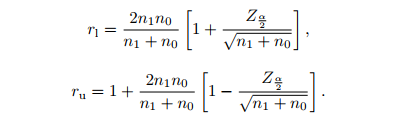例题
一个检定装置收到下述信号,能否说该信号是纯粹随机干扰?
给出检验问题:
$H_0:信号是存粹随机干扰, \leftrightarrow H_1:信号不是纯粹随机干扰$
在原假设成立的情况下,信号$X_i\sim B(1,p),p=\frac{n_1}{n}$
首先,计算基本的$n,n_0,n_1,R,E(R),var(R)$，然后计算$Z$，完事儿。
2.3.2 三类及多类游程检验
比如足球赛有输,赢,平局三种情况，假设一串游程有k个不同的值，有出现频数$n_1, n_2, ..., n_k, \sum_{i=1}^k n_i = n, p_i = \frac{n_i}{n}$，对付这种问题的方法也是使用近似正态，一般要求$n>12$的情况下可以通过$Z$的过大或者过小拒绝原假设。
\begin{aligned} E(R)&=n(1-\sum_{i=1}^k p_i^2)+1 \\ var(R)&=n[\sum_{i=1}^k(p_i^2-2p_i^3)+(\sum_{i=1}^k p_i^2))^2] \end{aligned}
2.4 Wilcoxon 符号秩检验
2.4.1 Wilcoxon符号秩统计量
前面介绍的统计推断仅仅依赖于数据符号，这类方法对连续分布的形态没有要求。Wilocoxon符号秩检验讨论对称分布，检验一个分布是否对称。
对于对称分布，

对称中心只有一个，但是中位数可能有很多个。
由于对称中心是中位数，因此在对称中心两侧应该大致各有一半左右的数据。
对称中心两侧数据分布密度相近。

对称中心

称连续分布$F(x)$关于$\theta$对称，如果$\forall x \in \mathbb{R}, F(\theta -x)=P(X<\theta-x)=P(X>\theta+x)=1-F(x=\theta)$

Wilcoxon符号秩统计量
先把数据取绝对值$|X_1|, |X_2|,..., |X_n|$，排序，得到绝对值顺序统计量$|X|_{(1)}, |X|_{(2)},..., |X|_{(n)}$。如果数据关于零对称，那么原来取正值的数据和原来取负值的数据应该均匀地落在这一绝对值顺序统计量序列中。
$W^+=\sum_{j=1}^n j W_j = \sum_{j=1}^nR_j^+ S(X_j)$
其中$R_j^+$是绝对值观测$|X_j|$，$S(X_j)$是符号示性函数，正为1，$W_j$就是与$|X|_{(j)}$对应的原样本点的示性函数$S(X_{D_j})$，$R_j^+S(X_j)$被称为符号秩统计量。
设某一组样本为$\{ 9, 13, -7, 10, -18, 4\}$，其绝对值秩$R_j^+$为$\{ 3, 5, 2, 4, 6, 1\}$，示性函数值为$\{ 1, 1, 0, 1, 0, 1\}$，矩阵乘法就得到$W^+=13$
下面有几个定理

 如果原假设$H_0:\theta = 0$成立，则$S(X_1), S(X_2), ..., S(X_n)$独立于$(R_1^+, R_2^+, ..., R_n^+)$.
 如果原假设$H_0:\theta = 0$成立，则$S(X_1), S(X_2), ..., S(X_n)$独立于$(D_1, D_2, ..., D_n)$. $D_j$是所谓的反秩，$|X_{D_j}|=|X|_{(j)}$.
 如果原假设$H_0:\theta = 0$成立，则$W_1,..., W_n$实际上同分布，$P(W_i=1)=P(W_i=1)=\frac{1}{2}$

2.4.2 Wilcoxon符号秩检验及抽样分布
检验流程
$H_0:M\leqslant M_0, \leftrightarrow H_1:M>M_0$

对所有的$i$，计算$|X_i-M_0|$，为样本点到$M_0$的距离
绝对值排序，得到新的秩，如果有结，取平均秩处理。
$W^+$等于$X_i-M_0>0$的$|X_i-M_0|$的秩和， $W^-$则等于$X_i-M_0<0$的$|X_i-M_0|$的秩和。二者之和$W^++W^-=n(n+1)/2$
估计
$W$统计量值，查表
决策

$W^+$在原假设下的精确分布
首先明确原假设下$W_j\sim b(1,0.5)$，那么
$Ee^{tjW_j}=\frac{1}{2}e^{tj}+\frac{1}{2}e^{0}=\frac{1}{2}(1+e^{tj})$
Wilcoxon符号秩统计量$W^+$的母函数
$M_n(t)=Ee^{tW^+}=Ee^{t\sum jW_j}= \Pi_{j=1}^n Ee^{tjW_j}=\frac{1}{2^n}\Pi_{j=1}^n(1+e^{tj})$
根据母函数的性质
$M_n(t)=P_{H_0}(W^+=0)+P_{H_0}(W^+=1)e^t\\+P_{H_0}(W^+=2)e^{2t}+...$
所以很容易得到$W^+$的分布概率，展开算就好。
大样本$W^+$分布
大样本下用精确算法太累，可以如下近似正态分布
$E(W^+)=E(\sum jW_j) =\frac{n(n+1)}{4}\\ V(W^+)=V(\sum jW_j)) = \sum j^2 E(W_j) = \frac{n(n+1)(2n+1)}{6}\frac{1}{4}$
自然可以构造$Z$，值得一提的是，

如果样本量较小，分子加一项$C$进行连续性修正

$C=0.5$如果$W^+>\frac{n(n+1)}{4}$，反之取$C=-0.5$

如果有结，分母减一项$\frac{\sum_{i=1}^g (\tau^3-\tau)}{48}$

Walsh平均值
$\{X_u':X_u'=\frac{x_i+x_j}{2},i\leqslant j, u=1,2,...,\frac{n(n+1)}{2}\}$
唯一要注意的就是，$i=j$的时候也要算哦！
之前使用的Wilcoxon符号秩统计量等价于Walsh平均值的正数计数，
$W^+= \# \{X_u'>0 \}$
H-L估计量
用来估计分布的对称中心
假设$X_1,X_2,...,X_n$独立同分布取自$F(x-\theta)$,$F$关于零点对称，定义$\theta$的Hodges-Lehmann点估计量为Walsh平均值的中位数
$\hat \theta = median\{ X_u'\}$
2.5 单组数据位置参数置信区间估计
2.5.1 基于顺序统计量
2.5.2 基于bootstrap
2.6 估计量的稳健性评估
新书
2.7 正态记分检验
正态记分检验是对于Wilcoxon符号秩检验的一个变型，把秩改成正态分布分位点，将依赖于秩的检验转换为对分位点大小的检验。同时，这个检验对于分布尾部数据做差距放大处理，而对中间数据做差距压缩处理，强调尾部数据对于位置判断的影响。
从Wilcoxon的检验问题开始，一个分布是否关于$M_0$对称：
$H_0: M=M_0\leftrightarrow H_1: M\neq M_0$
通过对于$|X_i-M_0|$的排序给出对应的秩$R_i$，给出符号正态记分
$s_i=\Phi^{-1}(\frac{n+1+R_i}{2n+2}) sgn(X_i-M_0)$
其中符号函数
sgn(X_i-M_0)= \left \{ \begin{aligned} 1, &X_i >M_0 \\ -1, &X_i
在观测值总体分布接近正态情况或者大样本情况下，如下正态记分检验统计量近似服从标准正态分布
$T=\frac{W}{\sqrt{\sum_{i=1}^n s_i^2}} \stackrel{\mathcal{L}}{\to} N(0,1)$
拒绝域和检验问题方向

如果备择假设为双边的，那么要计算标准正态分布下的双边尾概率$2P(x>|T|)$，越小越拒绝
如果是$H_1:M>M_0$，那么我们关注的是对称中心是否偏右很多，如果真实的对称中心在$M_0$右侧，这会导致$W$很大，因为大部分大秩的$s_i$都是正的。所以这时候我们的拒绝域是$\{T>z_{1-\frac{\alpha}{2}} \}$，或者算$p=P(x>T)=1-\Phi(T)$
如果是$H_1:M，相反，当$W$很小的时候会反映出真实的对称中心在$M_0$左侧，所以拒绝域是$\{T，或者算$p=P(x

2.8 分布的一致性检验
现实应用当中我们经常需要检验一组数据是否来自于另外一种分布，比如

一组连续性数据是否来自与正态分布
一组离散型数据是否来自与$Poisson$分布

2.8.1 $\chi^2$拟合优度检验
这个检验非常基础，就是看真是观测数$O_i$与期望观测数$E_i$是否吻合。假设$\forall p_i$是理论分布，
\begin{aligned} &H_0:总体分布为 \forall p_i,i=1,2,...,c \\ &H_1:总体分布不为 \forall p_i, i=1,2,...,c \end{aligned}
通过总量计算期望观测数$E_i=np_i，$定义Pearson$\chi^2$统计量
$\chi^2=\sum_{i=1}^c \frac{(O_i-E_i)^2}{E_i}=\sum_{i=1}^c \frac{O_i^2}{E_i}-2n+n$
$\chi^2$检验的拒绝域从来是统计量值越大的方向，因为统计量非负。这里拒绝域$W=\{\chi^2 > \chi^2_{\alpha, c-1}\}$，$c-1$是自由度
应用

均匀分布的$p_i$全等
泊松分布的$P(X=x)=e^{-\lambda} \frac{\lambda^x}{x!}$，而且其中的$\lambda$是泊松分布的期望，需要通过数据估计$\hat \lambda=\bar x$
检验正态性，将数据做离散化分组处理，搞出每一组的组件概率算期望频数

2.8.2 K-S正态性检验
这是几组非常著名的关于正态性的检验Kolmogorov-Smirnov,J-B,Liliefor
K-S方法的精神是以样本数据的累计频数分布和特定理论分布比较，如果相差很小，则支持推断该样本取自某特定分布族：
\begin{aligned} &H_0:样本所来自的总体分布服从某特定分布 \\ &H_1:样本所来自的总体分布不服从某特定分布 \end{aligned}
检验统计量$D=\max\limits_{1\leqslant i \leqslant n} |F_n(x_{(i)})-F_0(x_{(i)})|$中前者是随机样本的累积概率函数，后者是理论分布函数。
检验标准需要查表K-S D临界值(单一样本)，拒绝域为$D>D_{\alpha,n}$，单边。
2.8.3 Liliefor 正态分布检验
用样本均值$\bar x$和样本标准差$s$代替总体的期望$\mu$和标准差$\sigma$，然后使用K-S正态性检验法，统计量$D$查同一个表。
2.9 单一总体渐进相对效率对比
日后更新
问题列表

随机游程检验大样本情况下的$\frac{n_1}{n_0} \to \gamma$怎么理解
PDF P60也$Z$得公式有没有写错
怎么近似计算拒绝域的临界值$r_l,r_u$
分布一致性检验 有没有跟回归分析的类似
大作业怎么抽各个层的啊
母函$M_n(t)$数的性质


展开全文统计学 统计模型
• 1. χ2\chi^2χ2独立性检验的原理 若随机变量X,YX,YX,Y的分布函数分别为F1(x)和F2(y)F_1(x) 和 F_2(y)F1​(x)和F2​(y), 且联合分布为F(x,y)F(x, y)F(x,y), 则X与Y的独立性归结为假设检验问题： H0:F(x,y)
在单样本问题中, 人们想要检验的是总体的中心是否等于一个已知的值. 但在实际问题中, 更受注意的往往是比较两个总体的位置参数; 比如, 两种训练方法中哪一种更出成绩, 两种汽油中哪一种污染更少, 两种市场营销策略中哪种更有效等等.
1. $\chi^2$独立性检验的原理
若随机变量$X,Y$的分布函数分别为$F_1(x) 和 F_2(y)$, 且联合分布为$F(x, y)$, 则X与Y的独立性归结为假设检验问题：
$H_0: F(x,y)=F_1(x)F_2(y) \quad H_1: F(x,y)\neq F_1(x)F_2(y)$
若X与Y为分类变量，其中X的取值为$X_1, X_2,...,X_r$, Y的取值为$Y_1,Y_2,...,Y_s$, 将X与Y的各种情况组合用一张$r \times s$列联表表示，称为$r\times s$二维列联表，如下图所示：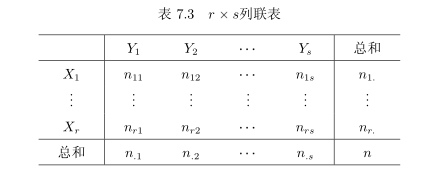表中$n_{ij}$表示n个随机试验的结果中X取$X_i$及Y取$Y_j$的频数，$\sum_{i=1}^r\sum_{j=1}^sn_{ij}=n$.
$n_{i.}=\sum_{j=1}^sn_{ij}, i=1,2,...,r, 表示各行之和$
$n_{.j}=\sum_{i=1}^rn_{ij}, j=1,2,...s, 表示各列之和$
令$p_{ij}=P(X=X_i, Y=Y_j), p_{i.}=P(X=X_i), p_{.j}=P(Y=Y_j), i=1,2,...,r; j=1,2,...,s,$ 则X与Y的独立性检验等价于下述检验：
$H_0: p_{ij} = p_{i.}p_{.j}, \forall 1\le i \le r, 1\le j \le s \quad H_1: \exist (i,j), p_{ij}\neq p_{i.}p_{.j}$
注: 若X与Y 为连续型随机变量, 这时将它们的取值范围分成r个及s个互不相交的小区间, 用$n_{ij}$ 表示n个随机试验的结果中“X属于第i个小区间, Y 属于第k个小区间”的频数 $(i=1,2,...,r; k=1,2,...,s)$ 这时可将X与Y 的独立性转化为列联表的独立性检验问题.
由于$p_{i.}$的极大似然估计为$\hat p_{i.} = n_{i.}/n$, $p_{.j}$的极大似然估计为$\hat p_{.j}/n$, 因此若$H_0$成立，则$p_{ij}$的极大似然估计为$\hat p_{i.} \hat p_{.j}=n_{i.}n_{.j}/n^2$. 从而X取代$X_i$, Y取代$Y_j$(试验数据落入第$(i,j)$个类)的理论频数为$n \times n_{i.}n_{.j}/n^2 = n_{i.}n_{.j}/n$. 由此构造检验统计量为：
$\chi^2=\sum_{i=1}^r\sum_{k=1}^s[n_{ij}-\frac{n_{i.}n_{.j}}{n}]^2/\frac{n_{i.}n_{.j}}{n}$
可以证明在原假设成立时， $\chi^2$近似服从$\chi^2((r-1)(s-1))$
2. 使用Python展示和计算
在python中的pandas库中有一个DataFrame的数据结构，可以用来保存二维数据，并进行计算。
例1： 对表1所示频数分布表，以59%显著水平，检验色觉与性别是否相关。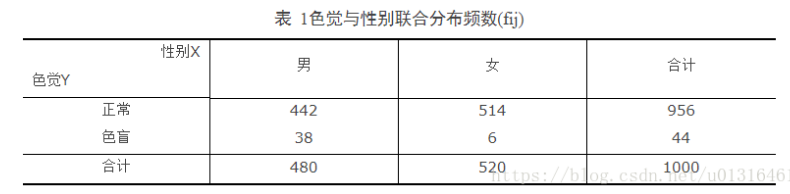2. 1.  初始化数据
import numpy as np
import pandas as pd
from scipy import stats
d=np.array([[442, 514],[38,6]])
r,s = d.shape
df = pd.DataFrame(d, columns=['male', 'female'], index=['normal', 'blindness'])

上述代码已将初始化数据存入df，显示如下：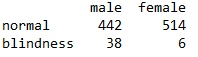2.2. 计算边际频数
首先在df基础上建立一个数据框（数据一致）。并在新建的数据框基础继续计算。
df1 = df[:]
df1= pd.DataFrame(df1)
df1['r_tot']=np.sum(df1,axis=1)
df1.loc['c_tot']=np.sum(df1, axis=0)

经过上面的处理，df1就是带有编辑频数的数据框。显示如下：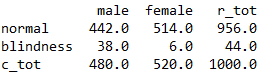2.3. 计算理论频数分布表
r_tot = df1['r_tot'][:-1]
c_tot=df1.loc['c_tot'][:-1]
total = np.sum(r_tot)
data = np.zeros((r,s))
for i in range(len(r_tot)):
for j in range(len(c_tot)):
data[i,j]=r_tot[i]*c_tot[j]/total
df2=pd.DataFrame(data, index=['normal', 'blindness'], columns=['male', 'female'])

至此，我们将计算得到的理论频数分布表保存在了df2中，而原始数据在df中，如下图所示：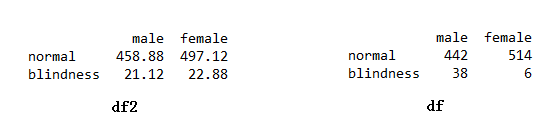2.4. 统计量计算
为了清晰整个计算过程，这里添加一步（对计算结果来说是多余的）产生统计量数据框的代码：
(df-df2)**2/df2

其产生的统计量数据框如下图：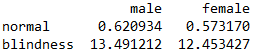其实，下面一行代码即可获得卡方统计量的值并获取pval：
chi_square=np.sum((df-df2)**2/df2).sum()
stats.chi2.sf(chi_squre, df=(r-1)*(s-1))

得到$\chi^2=27.13874340443378, pval=1.8936459120177876e-07$
因为$pval \lt \alpha=0.05$, 所以拒绝原假设，可以认为色盲和性别有关系


展开全文• 目录15.1 导言15.2 随机森林的定义15.3 随机森林的细节15.3.1 集外样本Out of Bag Samples15.3.2 变量重要15.3.3 邻近图Proximity Plots 15.1 导言 P587 委员会committee，只关注票数，平均并不重要。用于分类 P...
（总感觉random forest没有gradient boosting效果好，本章的实验图也有这种感觉）
目录15.1 导言15.2 随机森林的定义15.3 随机森林的细节15.3.1 集外样本Out of Bag Samples15.3.2 变量重要性15.3.3 邻近图Proximity Plots15.3.4 随机森林和过拟合15.4 随机森林的分析15.4.1 方差和去相关性De-Correlation的影响15.4.2 偏差15.4.3 自适应最近邻文献笔记
15.1 导言

P587 委员会committee，只关注票数，平均并不重要。用于分类
P587 随机森林random forest是对bagging的修改，建立一个去相关性de-correlated树的集合。在许多问题上，与boosting表现相似，而且训练和调参更简单

15.2 随机森林的定义

P587 树是bagging的理想模型，因为它们可以捕捉数据中复杂的交叉项，而且如果树足够深，会有相对较低的偏差
P588 注意，经过bagged的树的偏差和单个bootstrap树的偏差是一样的，因为bagged的树都是同分布的。唯一改善的是降低方差。这和boosting相反，boosting中树以自适应的方式减小偏差，因此不是同分布的
P588 如果$B$个变量是同分布i.d.，identically distributed但不是independent，有正的相关系数$\rho$，平均后方差为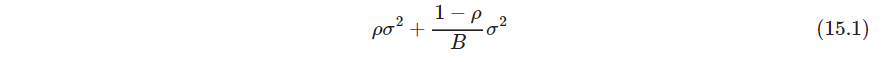见习题15.1，另外根据方差大于等于0，得到$\rho\ge -\frac{1}{B-1}$，也即相关稀疏不可能太小
随着$B$增大，第二项消失，第一项主导方差，随机森林的想法是通过降低树之间的相关系数来减小平均方差，同时又不使单个方差$\sigma^2$增大太多。（如果特征数量少了，单个方差会增大吗，我感觉是会的，比如考虑$y$和$x$的线性关系，$x$本身带噪声，多个$x$的观测得到的模型比单个$x$方差小。所以特征少了，方差可能大了，偏差可能也大。15.4节图15.10有分析）
可以通过在生成树的过程中对输入变量进行随机选择来实现，每次分割时，随机选$m\le p$个输入变量作为候选，一般地，取$m=\sqrt p$甚至$1$
P589 并非所有估计都可以通过这种震荡数据的方式来改善，似乎强非线性估计，比如树，改善最大。对于bootstrapped树，$\rho$一般很小，0.05或更小，见图15.9. 而$\sigma^2$不比原树的方差大（所以这里的$\rho$是实验做出来的，不是从数据推算的）
bagging不会改善线性的估计，比如样本均值的估计，或者样本方差，其bootstrapped均值之间成对相关系数大约50%，见习题15.4
P590 McNemar检验
P590，591 随机森林和GBM的一些实验对比
P592 Wilcoxon检验（没有细看）

15.3 随机森林的细节

P592 发明者给出两条推荐，对于特征数量为$p$，任务分类，随机选择特征数量$m$的默认值为$\left \lfloor \sqrt p \right \rfloor$，最小结点大小为$1$（应该是指结点中样本量）；对于分类，默认值为$\left \lfloor p/3 \right \rfloor$，最小结点大小为$5$

15.3.1 集外样本Out of Bag Samples

P592 随机森林一个重要的特征是它对集外out-of-bag,OOB样本的使用：对每个观测$z_i=(x_i, y_i)$，仅通过对部分树的平均来预测，这部分树是对应那些$z_i$不出现的. OOB误差几乎等价于通过$N$折交叉验证得到的估计，见习题15.2（其实也能理解，当树的数量$B$很大时，不包含$z_i$的那一堆树就可以看作是用把$z_i$排除掉的剩下的样本进行随机森林拟合产生的集合）
因此与许多其它的非线性估计器不同，随机森林可以逐步拟合，交叉验证沿着拟合进度逐步进行．一旦OOB误差稳定，训练可以终止（有些非线性估计器需要拟合完之后，才能算CV误差，不能边拟合边算）

15.3.2 变量重要性

P593 度量特征重要性的第一种方法：可以用和10.13节一样的方法Relative Importance构造变量重要性。通过比较图15.5和10.6，两者差不多。不过Boosting完全忽略了一些变量，而随机森林不会．切分候选变量增加了任意单个变量被包含进随机森林的概率
P593 第二种方法：随机森林也采用OOB样本来构造重要性度量，当生成第$b$棵树时，得到OOB样本，记录预测的准确度．接下来打乱第$j$个变量在OOB样本中的顺序，再一次计算准确度．对所有的树应用这种随机打乱，计算平均降低的准确度，然后用作对随机森林中第$j$个变量的重要性的度量. （weiya注：这种方法得到的重要性也称为Permutation Importance。sklearn分析了这两者不同）
尽管这两种方法得到的特征排名类似，图15.5说明Permutation Importance在变量间更加均匀．
随机化有效取消了单个变量的影响，这很像在线性模型中设置对应变量的系数为0，见习题15.7
这个方法不能度量当某个变量不可用时，对结果的影响。因为如果重新拟合，其他变量可能代替之

15.3.3 邻近图Proximity Plots

P595 积累训练数据的$N\times N$的邻近矩阵Proximity matrix（回顾14.3.1节），对每棵树，任意一对OOB观测值如果共享一个叶子，则邻近proximity加1. 邻近矩阵进一步可用多维缩放至2维。尽管原始数据可能是高维的、包含混合变量的，但邻近图给出随机森林看来彼此充分靠近的观测（这里用的是相似性，MDS中用的是距离，这两者不知道中间是怎么转换的）
P595 不管什么数据，随机森林的邻近图经常看起来非常类似，这也让人怀疑其效用. 图趋向于星形，每个类别一个臂，当分类效果越好，图就会越清楚

15.3.4 随机森林和过拟合

P596 当特征数量增多，而有效特征的比例变小时，随机森林会在随机选的特征数量$m$小的情况下表现不好
当相关变量个数变多，随机森林的表现在噪声变量增加时出奇地鲁棒。与boosting相比，这不会损害随机森林的表现．这种鲁棒性大部分是因为误分类代价相对于每棵树中概率估计的偏差和方差的不敏感性（这一大段不理解。分类问题的方差是普遍比回归问题要小吗？）
P596 随机森林中树数$B$增大，不会使随机森林序列过拟合。
然而充分生长的树的平均可以导致模型太丰富too rich，产生额外方差. Segal (2004)通过控制单个生成树的深度小幅度改善效果．本书作者的经验是采用充分生长的模型也不会有太大代价，并且少调一个参数

15.4 随机森林的分析

P597 本节集中讨论回归和平方误差损失，而0-1损失下的偏差和方差都会更复杂，参考7.3.1节

15.4.1 方差和去相关性De-Correlation的影响
（这里de-correlation就是指各个树之间通过只使用部分特征减小预测值相关系数）

P597 方差的极限形式$B\to \infty$是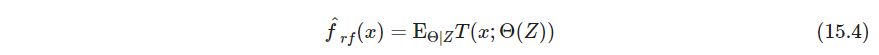其中$\bm Z$是训练数据集。由式15.1得到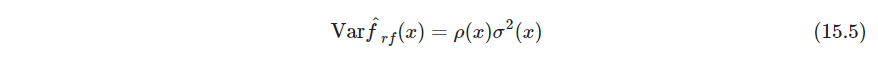其中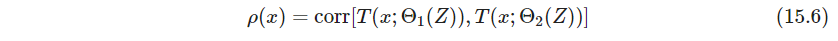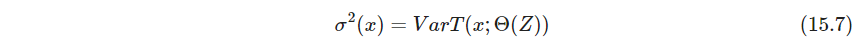式15.6和15.7中计算的波动是在$\bm Z$的条件下的，由自助采样和特征采样造成；以及$\bm Z$自身的采样波动（所以训练集$\bm Z$自身也会存在波动，$\bm Z$采样完了之后再采样$\Theta_1,\Theta_2$）（这里$\bm Z$应该确实是训练集，没有经过bootstrap。如果$\bm Z$表示的是bootstrap之后的，考虑用所有特征拟合树的bagging情况，必有$\rho(x)=1$，这显然不是我们想研究的）
在x处拟合的成对树的条件协方差$Cov_{\Theta_1|\bm Z,\Theta_2|\bm Z}[T(x;\Theta_1(\bm Z), T(x;\Theta_2(\bm Z))]=0$，这是必然的，因为$\Theta_1|\bm Z,\Theta_2|\bm Z$独立。由习题15.5（这里需要条件方差公式Law of total covariance，式15.9也需要）图15.9做了个实验，考察$m$对相关系数的影响
考虑方差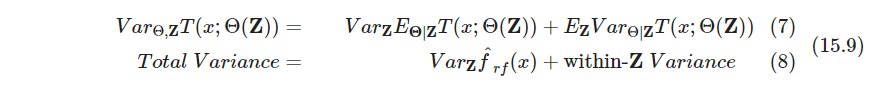（这里很有趣的是式(15.9)第一项就是随机森林的Variance，而总方差是单棵树的方差。15.5给出了这两者的另一个关系式。这两个式子看起来是等价的。这三个量的关系怎么看都觉得很神奇）
单棵树的方差在$m$的大部分取值不会明显改变，不过$\rho^2$变得多，所以随机森林的总方差在$m$小时显著降低（也就是图15.10右图所示）

15.4.2 偏差

P600 和bagging中一样，随机森林的偏差与任意单棵采样后的树$T(x;\Theta(\bm Z))$一样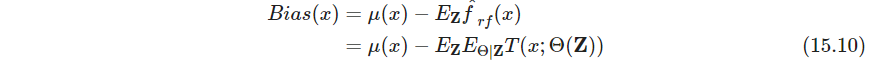因为随机化特征和减小的样本空间的约束，这一般比由$\bm Z$生成的未剪枝的树的偏差的绝对值要大。因此bagging或随机森林带来的改善仅仅是方差降低的结果
P601 随机森林与岭回归的相似性．对于大量的特征，通过岭回归正则化能允许所有的变量都有它们的影响，尽管被削弱．$m$较小的随机森林表现出相似的平均．每个相关的变量都会成为主分割，并且平均降低了任一单个变量的贡献．
（模拟例子15.8，用岭回归达到最优结果的约为$df(\lambda_{opt})\approx 29$。为什么这么低，明明50个变量都起作用？是不是噪声越大，参数有效数越低）

15.4.3 自适应最近邻

P601 随机森林分类器是kNN分类器的加权版本。当每棵树长到最大时，对特定$\Theta^*$， $T(x;\Theta^*(\bm Z))$是其中一个训练样本的响应值。这里忽略了一个叶子结点多个同类的情况。平均的过程是对训练响应变量赋予权重，最终对预测值进行投票．那些靠近目标点的观测赋予了权重，相当于一个等价核，这些结合在一起形成了分类边界

文献笔记

P602 Dietterich(2000b)在每个结点出对前20个候选分离排序，接着随机从中选择，从而提升bagging性能
P602 Friedman and Hall (2007)证明了不放回子采样能有效代替bagging. 在大小为$N/2$的样本上对树的生长和平均近似等于bagging——在考虑偏差及方差的情况下；而采用更少的样本则会降低更大的方差——通过去相关处理

参考文献：
 Trevor Hastie, Robert Tibshirani, Jerome Friedman. The Elements of Statistical Learning, Second Edition
 ESL CN


展开全文机器学习 集成学习 bagging bootstrap
• RANSAC算法的基本假设是样本中包含正确数据(inliers，可以被模型描述的数据)，也包含异常数据(outliers，偏离正常范围很远、无法适应数学模型的数据)，即数据集中含有噪声。这些异常数据可能是由于错误的测量、错误...
转载请注明出处：

算法简介

RANSAC算法的基本假设是样本中包含正确数据(inliers，可以被模型描述的数据)，也包含异常数据(outliers，偏离正常范围很远、无法适应数学模型的数据)，即数据集中含有噪声。这些异常数据可能是由于错误的测量、错误的假设、错误的计算等产生的。同时RANSAC也假设，给定一组正确的数据，存在可以计算出符合这些数据的模型参数的方法。

基本思想描述

RANSAC基本思想描述如下：

①考虑一个最小抽样集的势为n的模型(n为初始化模型参数所需的最小样本数)和一个样本集P，集合P的样本数#(P)>n，从P中随机抽取包含n个样本的P的子集S初始化模型M；

②余集SC=P\S中与模型M的误差小于某一设定阈值t的样本集以及S构成S*。S*认为是内点集，它们构成S的一致集(Consensus Set)；

③若#(S*)≥N，认为得到正确的模型参数，并利用集S*(内点inliers)采用最小二乘等方法重新计算新的模型M*；重新随机抽取新的S，重复以上过程。

④在完成一定的抽样次数后，若未找到一致集则算法失败，否则选取抽样后得到的最大一致集判断内外点，算法结束。

以上内容摘自百度百科

下面用自己的理解说一下RANSAC

首先，RANSAC是一种估计系统模型的算法，既然是算法，我们需要搞清楚输入和输出是什么。RANSAC的

输入：一堆样本（观测数据）和系统模型

输出：适用于这堆样本的系统模型最优参数

算法实现过程：

符号说明：

k:算法随机抽样检验次数

n:确定模型参数需要的最少样本数

p:输入样本总数

M:初始化的模型参数

pinlier每次迭代初始化的内点

M*：单次抽样检测优化后的模型参数

M**：k次抽样优化的系统最优模型参数

RANSAC会有若干次抽样检测，次数由k决定，每一次抽样检测是为了得到M*，最后从k个M*中去最优的M*作为M**。

单次抽样检测步骤如下：

1 随机选取n个样本作为pinlier，用pinlier计算一个初始模型参数M.

2 用M检查所用剩余样本，符合模型M的样本认为是内点，否则是外点。

注：符合模型的含义是与模型的误差小于一定值。

3 把所有的内点，采用最小二乘法重新优化系统模型参数得到M*。

抽样k次，选择最优的M*作为M**。

注：最优的含义是此模型参数使划分的局内点个数最多

如果迭代k次，最优模型参数划分的局内点太少，认为此次算法失败。

以上就是RANSAC的一般流程。本质上，RANSAC就是最小二乘，随机了k次最小二乘，每次最小二乘之前，去掉一些本次估计认为的外点，只对内点进行最小二乘。

一个简单的例子

我们举个简单的例子-直线拟合，来说明一下，在具体应用中，RANSAC的参数含义。

下图是用于拟合直线含噪声的样本点，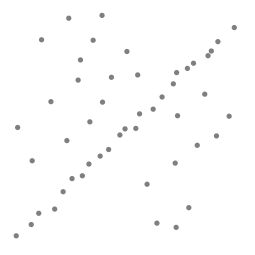上述的参数，在直线拟合具体问题中，就变成了

k:算法随机抽样检验次数

n:确定模型y=ax+b中参数a，b需要的最少样本数，这里是至少两对点，因此n=2。

p:输入点的总数

M:初始化的a，b参数

pinlier：每次迭代初始化的内点

M*：单次抽样检测优化后的a，b。

M**：k次抽样优化的系统最优a，b。

RANSAC比直接最小二乘的优点是对噪声鲁棒性较强。直线拟合效果如下：

左图为最小二乘拟合，右图为RANSAC估计效果。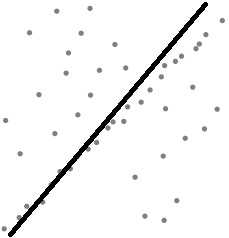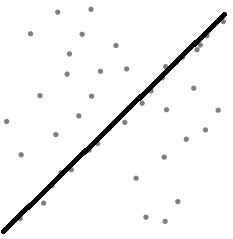展开全文RANSAC
• 在统计学中，差异显著性检验是“统计假设检验”（Statistical hypothesis testing）的一种，用于检测科学实验中实验组与对照组之间是否有差异以及差异是否显著的办法。 在实验进行过程中，尽管尽量排除随机误差的...假设检验 非参数检验 Scipy 差异分析
• Fligner-Policello 是对具有连续累积分布的两个组合随机变量的非参数检验。 它是对既不假定正态也不假定方差相等（Behrens-Fisher 问题）的群体的处理效果的稳健排序检验。 它做了一个假设，当分布是对称的时它是...matlab
• Fisher精确检验（基于超几何分布）的基本假设可以通俗理解为：如果当前分组方式是一种随机现象（无意义的分组），那么基于当前分组出现当前离散分布的可能有多大（当然严格意义上应该这么说：比当前分布状态更极端...
• H=GTEST(X,ALPHA) 执行 Geary 检验以确定复合正态 PDF 的原假设是否是关于具有所需显着水平 ALPHA 的随机样本 X 的总体分布的合理假设。 H表示根据条件语句的MATLAB规则进行假设检验的结果： H=1 => 不要在显...matlab
• 现有随机性检测规范由于没有系统地讨论统计检验和随机本质的联系，难以指导实际的安全性评估工作。通过随机性的不可区分性定义，论证了随机性检测在理想情况下需要考察所有概率多项式时间算法的区分情况，因此对随机...
• 检验需要符合随机分布的假定，也就是说，两组个案数据间的差异无其他人为的影响因素。 需要注意的是，“独立样本T检验检验的是两组个案，而不是两个变量，因此需要构建个案组数据；另外，其分组变量需用数值...
• H=CHI2TEST(X,ALPHA) 执行 Pearson 卡方检验的特殊情况，以确定复合正态 PDF 的原假设是否是关于具有所需显着水平 ALPHA 的随机样本 X 的总体分布的合理假设。 H表示根据条件语句的MATLAB规则进行假设检验的...matlab
• 显著性检验（significance test）就是事先对总体（随机变量）的参数或总体分布形式做出一个假设，然后利用样本信息来判断这个假设（备择假设）是否合理，即判断总体的真实情况与原假设是否有显著性差异。或者说，...
• 先概括一下：本文主要阐述了A/Btest中组间差异的比率检验（单比率检验，双比率检验），统计功效和，以及何通过显著水平还有统计功效反实验所需选样本量。根绝这些理论使用python对着三个功能封装成类，进行实现 一...ab测试 python
• 假设检验中有时会出现样本点落在接受域与拒绝域的边界上,已有的方法是用随机检验来处理。通过 对随机检验的研究,发现了其中可能导致显著水平改变较大。根据心理学中韦伯-费希纳定律,从而通过引 入显著水平的...
• 推断统计部分（一）—样本与分布的关系及其检验统计量标签（空格分隔）： 概率论与数理统计统计除了可以描述随机变量特征之外，还有一个重要作用，推断！这也是为什么把统计分为描述统计和推断统计的原因，以...
• 独立样本t检验用于比较独立设计（也叫完全随机设计）的两组总体均数是否相同。一般而言此法根据分组设计而决定，但有时候也可以按某个定量变量的截断值（cut point）分成两组，比较这两组某个变量的均数。例如，一家...
• 传送：随机变量概率分布函数汇总-离散型分布+... 假设检验-单样本t检验  假设检验-两服从正态分布的独立总体均值检验 一、单样本方差检验-需服从正态分布  chisq.var.test=function(x,var,mu=Inf,altern...假设检验 方差检验
• 2. 背景知识2.1 真随机和伪随机概念根据密码学原理，要想对一个“随机数”进行随机性检验有以下几个标准：统计学伪随机性 - 在给定的随机比特流样本中，1 的数量大致等于 0 的数量，也就是说，“10”...
• 输入：n比特序列样本Z，显著水平α 输出：检验通过或检验失败 步骤：（参见文的5.4.4节） 1. 在此序列截断中统计比特“0”和“1”的个数，分别记为和。 2. 计算检验统计量 （该检验统计量服从自由度为...随机数
• H = MTEST(X,ALPHA) 执行 Smirnov-Cramer-Von Mises 检验的特殊情况，以确定复合正态 CDF 的原假设是否是关于具有所需显着水平 ALPHA 的随机样本 X 的总体分布的合理假设。 Smirnov-Cramer-Von Mises 检验基于...matlab
• 一组随机样本数据需要进行分析处理时，往往需要用到假设检验，对于离散变量discrete多用卡方检验，连续变量continuous用t检验或wilcoxon秩序和检验，具体的的使用场景如下 离散变量-卡方检验-适用条件 四格表： 所有...
• 假设检验就是事先对总体(随机变量)的参数或总体分布形式做出一个假设，然后利用样本信息来判断这个假设是否合理。 在验证假设的过程中，总是提出两个相互对立的假设，把要检验的假设称作原假设，记作H0，把与H0...python 机器学习 数据分析 人工智能 算法
• 显著性检验（significance test）就是事先对总体（随机变量）的参数或总体分布形式做出一个假设，然后利用样本信息来判断这个假设（备则假设）是否合理，即判断总体的真实情况与原假设是否有显著性差异。或者说，......